# KSEEB Solutions for Class 8 Maths Chapter 3 Axioms, Postulates and Theorems Additional Questions

Students can Download Maths Chapter 3 Axioms, Postulates and Theorems Additional Questions and Answers, Notes Pdf, KSEEB Solutions for Class 8 Maths helps you to revise the complete Karnataka State Board Syllabus and score more marks in your examinations.

## Karnataka State Syllabus Class 8 Maths Chapter 3 Axioms, Postulates and Theorems Additional Questions

Question 1.
Choose the correct option
(i) If a = 6 and b = a, then b = 60 by _______
A. Axiom 1
B. Axiom 2
C. Axiom 3
D. Axiom 4
Solution:
A. Axiom 1

(ii) Given a point on the plane, one can draw ________
A. unique
B. two
C. finite numberd
D. infinitely many
Solution:
(D) Infinetly many lines through that point.

(iii) Given two points in a plane, the number of lines which can be drawn to pass through these two points is ________
A. Zero
B. exactly one
C. at most one
D. more than one
Solution:
(B) Exactly one

(iv) If two angles are supplementary, then their sum is
A. 90°
B. 180°
C. 270°
D. 360°
Solution:
(B) 180°

(v) The measure of an angle which is 5 times its supplement is _________
A. 30°
B. 60°
C. 120°
D. 150°
Solution:
(D) 150°Question 2.
What is the difference between a pair of supplementary angles and a pair of complementary angles?
Solution:
If the sum of two angles is 180° then they are supplementary angles. If the sum of two angles is 90° then they are complementary angles.

Question 3.
What is the least number of non – collinear points required to determine a plane?
Solution:
Three (3)

Question 4.
When do you say two angles are adjacent?
Solution:
Two angles are said to be adjacent angles if they have a common vertex and a common side.

Question 5.
Let $$\overline{\mathbf{A B}}$$ be a segment with C and D between them such that the order of points on the segment is A, C, D, B suppose AD = BC prove that AC = DB
Solution: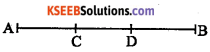AD – CD = BC – CD [Axiom 3]
AC = DB [In the fig AC – CD = AC , BC – CD = DB]Question 6.
Let $$\overrightarrow{\mathbf{A B}}$$ and $$\overrightarrow{\mathbf{A B}}$$ be two straight lines intersecting at O. Let $$\overrightarrow{\mathbf{O X}}$$ be the bisector of ∠BOD. Draw $$\overrightarrow{\mathbf{O Y}}$$ between $$\overrightarrow{\mathbf{O D}}$$ and $$\overrightarrow{\mathbf{O A}}$$ such that $$\overrightarrow{\mathbf{O Y}}$$ ⊥ $$\overrightarrow{\mathbf{O B}}$$ prove that $$\overrightarrow{\mathbf{O Y}}$$ bisects ∠DOA .
Solution:Construction: Produce [/latex] such that $$\overrightarrow{\mathbf{X O}}$$ to A point Z such that [/latex] such that $$\overrightarrow{\mathbf{O Z}}$$.is between [/latex] such that $$\overrightarrow{\mathbf{O A}}$$ and [/latex] such that $$\overrightarrow{\mathbf{O C}}$$
Proof : ∠AOZ = ∠BOX [vertically opposite angles]
∠BOX = ∠POX [$$\overrightarrow{\mathbf{OX}}$$ bisects ∠DOB]
∴ ∠AOZ = ∠DOX  [Axiom 1] … (i)
[ZOY = [YOX r=90°] … (ii)
(ii) – (i) ∠ZOY – ∠AOZ = ∠YOX – ∠DOX ∠AOY = ∠YOD [Axiom 3]
∴ [/latex] such that $$\overrightarrow{\mathbf{O Y}}$$ bisects ∠AOD

Question 7.
Let $$\overrightarrow{\mathbf{A B}}$$ and $$\overrightarrow{\mathbf{C D}}$$ be two parallel lines and $$\overrightarrow{\mathbf{P Q}}$$ be a transversal. Let $$\overrightarrow{\mathbf{P Q}}$$ intersect $$\overrightarrow{\mathbf{A B}}$$ in L. Suppose the bisector of ∠ALP intersect CD in R and the bisector of ∠PLB intersect CDin S prove that ∠LRS + ∠RSL = 90°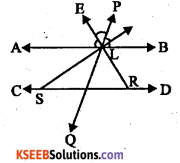Solution:
∠ALP + ∠BLP = 180° ∠Linear pair]
$$\frac{1}{2}$$ ∠ALP + $$\frac{1}{2}$$ ∠BLP = $$\frac{1}{2}$$180°
[Multiplying by $$\frac{1}{2}$$ ]
∠ELP + ∠FLP = 90° [∴ $$\overrightarrow{\mathbf{E L}}$$ and $$\overrightarrow{\mathbf{F L}}$$ are bisectors of ∠ALP and ∠BLP ]
∠ELF = 90°
[SLR = ∠ELF = 90°
[vertically opposite angles]
In ∆ SLR, ∠SLR + ∠LRS + ∠RSL = 180° [sum of the angles of the triangle is 180° ]
90 + ∠LRS + ∠RSL = 180°
∠LRS + ∠SL = 180 – 90
∠LRS + [RSL = 90°Question 8.
In the adjoining figure $$\overrightarrow{\mathrm{AB}}$$ and $$\overrightarrow{\mathrm{CD}}$$ are parallel lines. The transversals $$\overrightarrow{\mathrm{PQ}}$$ and $$\overrightarrow{\mathrm{RS}}$$ intersect at U on the line $$\overrightarrow{\mathrm{AB}}$$. Given ∠DWU = 110° and ∠CVP = 70° find the measure of ∠QUS.
Solution:∠ AUV – ∠CVP = 70°
[corresponding angles]
∠QUB – ∠AUV = 70° [vertically opposite angles]
∠BUW + ∠DWU = 180° [Interior angles on the same side of transversal]
∠BUW + 110°= 180°
∠BUW = 180-110° = 70°
∠BUW = ∠AUS= 70° [vertically opposite angles]
∠AUS + ∠SUQ + ∠QUB = 180° ∠AUB is a straight angle]
70 + ∠SUQ + 70 = 180°
∠SUQ + 140° = 180°
∠SUQ = 180° – 140°
∠SUQ = 40°Question 9.
What is the angle between the hours hand and minutes hand of a clock at (i) 1.40 hours (ii) 2.15 hours [use 1° = 60 minutes]
Solution:
i. 1.40 hours – 190°
ii. 2.15 hours – 22° 30′

Question 10.
How much would hour’s hand have moved from its position at 12 noon when the time is 4.24 p.m?
Solution:
144°

Question 11.
Let $$\overrightarrow{\mathrm{AB}}$$ be a line segment and let C be the midpoint of $$\overrightarrow{\mathrm{AB}}$$ Extend AB to D such that B lies between A and D. Prove that AD + BD = 2CD
Solution: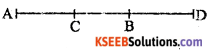= AC + CB + BD + BD [∵ AD = AC + CB + BD]
= CB + CB + BD + BD
[∴ AC = CB]
= CB + BD + CB + BD
= CD + CD
= 2CD = RHS
∴ AD + B D = 2CDQuestion 12.
Let $$\overrightarrow{\mathbf{A B}}$$ and $$\overrightarrow{\mathbf{C D}}$$ be two lines . intersecting at a point O. Let $$\overrightarrow{\mathbf{O X}}$$ be a ray bisecting [BOD. Prove that the extension of $$\overrightarrow{\mathbf{O X}}$$ to the left of O bisects ∠AOC.
Solution: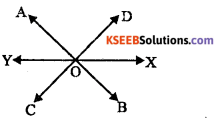∠DOX = ∠BOX [ ∵$$\overrightarrow{\mathbf{O X}}$$ bisects ∠BOD]
∠DOX =∠COY [vertically opposite angles] ….(i)
∠BOX = ∠AOY
[vertically opposite anglesj … (ii)
From (i) and (ii)
[vertically opposite] …(ii)
∠AQY – ∠COY [Axiom 1]
∴ $$\overrightarrow{\mathbf{O X}}$$ bisects ∠AOC

Question 13.
Let $$\overrightarrow{\mathbf{O X}}$$ be a ray and let $$\overrightarrow{\mathbf{O A}}$$ and $$\overrightarrow{\mathbf{O B}}$$ be two rays on the same side of $$\overrightarrow{\mathbf{O X}}$$ with $$\overrightarrow{\mathbf{O A}}$$ between $$\overrightarrow{\mathbf{O X}}$$ and $$\overrightarrow{\mathbf{O B}}$$ . Let $$\overrightarrow{\mathbf{O C}}$$ be the bisector of ∠AOB prove that ∠XOA + ∠XOB = 2∠XOC
Solution:
∠XOA + ∠XOB = 2∠XOCQuestion 14.
Let $$\overrightarrow{\mathbf{O A}}$$ and $$\overrightarrow{\mathbf{O B}}$$ be two rays and let $$\overrightarrow{\mathbf{O X}}$$ be a ray between $$\overrightarrow{\mathbf{O A}}$$ and $$\overrightarrow{\mathbf{O B}}$$ such ∠AOX > ∠XOB . Let $$\overrightarrow{\mathbf{O C}}$$ be the bisector of ∠AOB prove that ∠AOX – ∠XQB = 2∠COX
Solution: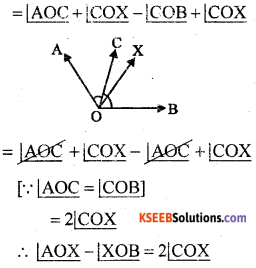Question 15.
Let $$\overrightarrow{\mathbf{OA}}$$, $$\overrightarrow{\mathbf{O B}}$$, $$\overrightarrow{\mathbf{OC}}$$ be three rays such that $$\overrightarrow{\mathbf{OC}}$$ lies between $$\overrightarrow{\mathbf{OA}}$$ and $$\overrightarrow{\mathbf{O A}}$$. Suppose the bisectors of ∠AOC and ∠COB are perpendicular to each other. Prove that B, O. A are . collinear.
Solution:
∠XOC + ∠YOC – 90° [data]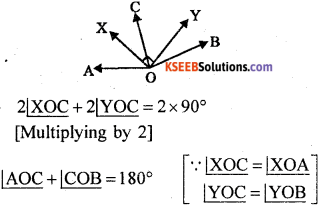∠AOB = 180°
A, O and B are collinear.Question 16.
In the adjoining figure $$\overrightarrow{\mathrm{AB}}$$ || $$\overrightarrow{\mathrm{DE}}$$ . Prove that ∠ABC – [DCB + [CDE = 180°
Solution:
Construction: Through C draw $$\overrightarrow{\mathrm{XY}}$$ || $$\overrightarrow{\mathrm{AB}}$$
Proof : ∠ABC + ∠BCX = 180° [sum of interior angles on the same side of trnasversal]
∴ ∠BCX = 180 – ∠ABC
∠CDE + ∠DCY – 180° [sum of interior angles on the same side of transversal]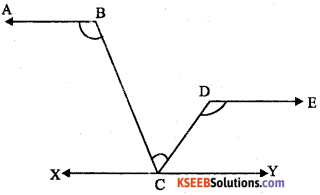∴ ∠DCY = 180° – ∠CDE
∠BCX + ∠BCD + ∠DCY = 180° [∵ XCY is straight angle]
180 – ∠ABC + ∠BCD + 180 – ∠CDE = 180° [By substituting]
360 – ∠ABC + ∠BCD – ∠CDE – 180°
360 – 180 – ∠ABC – ∠BCD + CDE
180° = ∠ABC – ∠BCD + ∠CDE
or
∠ABC – ∠BCD + ∠CDE = 180°

Question 17.
Consider two parallel lines and a transversal among the measures of 8 angles formed how many distinct numbers are there?
Solution:
There will be two distinct numbers.error: Content is protected !!